# Quiz 12: The Costs of Production

Business

Accounting profit: Accounting profit refers to the financial gain that is difference between total cost and total revenue. A person spends \$10 to prepare lemonade and sells at \$60; hence, the accounting profit of the person is as follows:The accounting profit is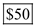. Economic profit: Economic profit refers to the additional profit that exceeds the opportunity cost. At the same time, if the person had mowed his neighbor's lawn, the might have earned \$40. Hence, the economic profit is as follows: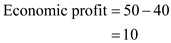The economic profit is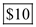. Hence, option 'a' is correct.

Opportunity cost : In economics, the opportunity cost refers to the benefits given up by an individual or business when an alternative is chosen over another. Fixed cost: It refers to the constant cost of production which remains same irrespective of the change in the level of output. Variable cost : It refers to the cost change with the change in the level of output. It is high at the higher level of outputs and low at the lower level of outputs. The sum of fixed cost and variable cost is equal to the total cost. Marginal cost : It is the additional cost of doing an additional activity. Average total cost : It results when the total cost is divided by units of quantity produced. a. The amount given up in order to take an action is equivalent to the amount of next best alternative. This is the opportunity cost of the action chosen. Hence, the first blank is filled with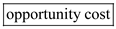. b. When marginal cost is below than average cost, the average cost tends to fall. When the marginal cost is above than the average cost, the average cost tends to rise. Hence, the second blank is filled with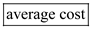. c. The cost remains fixed whether the quantity produced increases or decreases is said to be a fixed cost. For instance, Plant and machinery cost, installation cost, rent, insurance premium et cetera. Hence, the third blank is filled with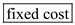. d. The cost increase with the increase in the number of units produced is said to be a variable cost. This is dependent on the quantity produced. For instance, labor cost, material cost et cetera. In the short-run, in the ice cream industry, cream and sugar are intermediate goods used in the production of ice creams. As the demand for ice cream increases, the demand for cream and sugar increases and thus the industry must spend more on these goods. Therefore, the cost of cream and sugar increases with the increases in the output of ice creams, this implies the cost of these goods are variable costs. Hence, the fourth blank is filled with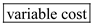. e. The gap between the total revenue and total cost is equal to the profit. Hence, the fifth blank is filled with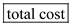. f. The cost spends on the additional unit of output produced is called marginal cost. Hence, the sixth blank is filled with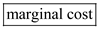.

Total revenue, total cost, and profit are interrelated. Economists assume that the goal of a firm is to maximize profit. Profit is a firm's total revenue minus its total cost. Total revenue is the amount that the firm receives for the sale of its output. Total cost is the amount that the firm pays to buy inputs. Thus, in order to determine the profit of a firm, one should know the total revenue and the total cost. Therefore, they are interrelated.